# Cofunction Identities

The cofunction identities show the relationship between sine, cosine, tangent, cotangent, secant and cosecant. The value of a trigonometric function of an angle equals the value of the cofunction of the complement. Recall from geometry that a complement is defined as two angles whose sum is 90°.

For example: Given that the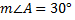the complement of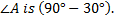Sine and cosine are cofunctions and complements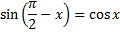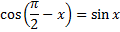Tangent and cotangent are cofunctions and complements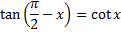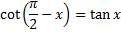Secant and cosecant are cofunctions and complements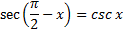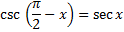Degree

 Sine and cosine are cofunctions and complements sin(90° - x) = cos x cos(90° - x) = sin x Tangent and cotangent are cofunctions and complements tan(90° - x) = cot x cot(90° - x) = tan x Secant and cosecant are cofunctions and complements sec(90° - x) = csc x csc(90° - x) = sec x

Degree Example:sin A =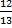cos B =A =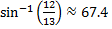B =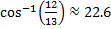cos(90° - A) = sin A

Using substitution:cos(90° - 67.4°) = sin 67.5°

cos(22.6°) = sin(67.5)°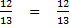Therefore, the value of cosine B is equal to sine A which is the cofunction and complement of B. The process remains the same whether you are in degree mode or radian mode.

Let's see how this can be applied.

Use the cofunction identities to evaluate the expression without a calculator!

sin2 (23°) + sin2 (67°)

Step 1: Note that 23° + 67° = 90° (complementary)

Step 2: use the cofunction identity and let x = 23°sin(90° - x) = cos x

therefore sin(67°) = cos(23°)

Step 3: use substitution sin2(23°) + cos2(23°)

Step 4: use the Pythagorean identity sin2θ + cos2θ = 1sin2(23°) + cos2(23°) = 1

Therefore, sin2(23°) + sin2(67°) = sin2(23°) + cos2(23°) = 1

 Related Links: Math Trigonometry Sum and Difference of Angles Identities Trigonometric Identities - Reciprocal Identities

To link to this Cofunction Identities page, copy the following code to your site: